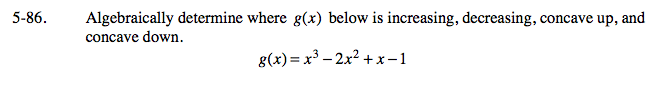### Home > CALC > Chapter 5 > Lesson 5.2.3 > Problem5-86

5-86.
1. Algebraically determine where g(x) below is increasing, decreasing, concave up, and concave down. Homework Help ✎

g(x) = x3 − 2x2 + x − 1The function f(x) is increasing when f '(x) > 0, and it is decreasing when f '(x) < 0.
The function is concave up when f ''(x) > 0, and it is concave down when f ''(x) < 0.